Select Page

# Solutions for MCQ CBSE 12 Science Maths Vector Algebra in English

Solutions for MCQ CBSE 12 Science Maths Vector Algebra in English to enable students to get Solutions in a narrative video format for the specific question.

Expert Teacher provides Solutions for MCQ CBSE 12 Science Maths Vector Algebra through Video Solutions in English language. This video solution will be useful for students to understand how to write an answer in exam in order to score more marks. This teacher uses a narrative style for a question from Vector Algebra not only to explain the proper method of answering question, but deriving right answer too.

Please find the question below and view the Solution in a narrative video format.

Question:

Solution Video in English:

You can select video Solutions from other languages also. Please check Solutions in ( Hindi )

## Similar Questions from CBSE, 12th Science, Maths, Vector Algebra

Question 1 : Find the direction of the vector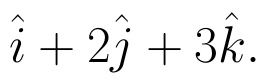(View Answer Video)

Question 2 : Find the projection of the vector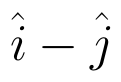on the vector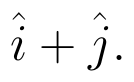(View Answer Video)

Question 3 : Find |a| and |b|, if (a + b).(a - b) = 8 and |a| = 8|b|. (View Answer Video)

Question 4 : Find the vector quantities :
(i)     Work                     (ii)      Force

Question 5 : Find |x|, if for a unit vector a,(x - a).(x + a) = 12. (View Answer Video)

### Inverse Trigonometric Functions

Question 1 : Write the value of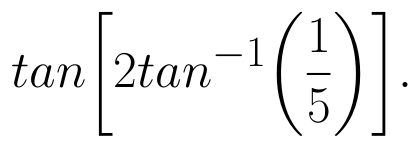(View Answer Video)

Question 2 : If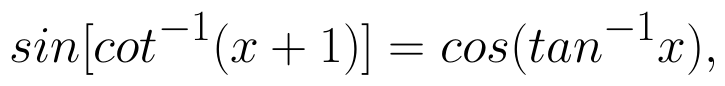then find x. (View Answer Video)

Question 3 : Find the principal value of sin-1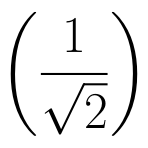. (View Answer Video)

Question 4 : If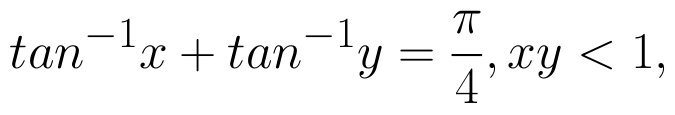then write the value of x + y + xy. (View Answer Video)

Question 5 : Write the principal value of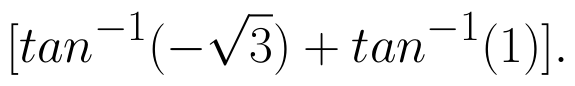(View Answer Video)

### Matrices

Question 1 : Find the transpose of the matrix: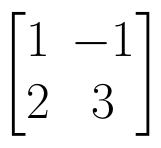. (View Answer Video)

Question 2 : Let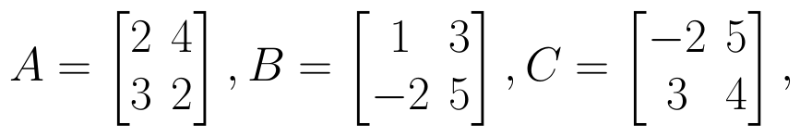Find 3A - C. (View Answer Video)

Question 3 : Find the value of x from the equation: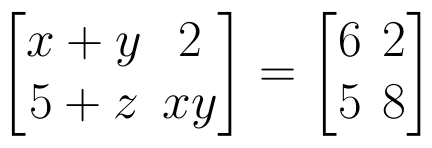. (View Answer Video)

Question 4 : Find the value of Y, if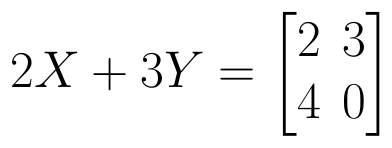and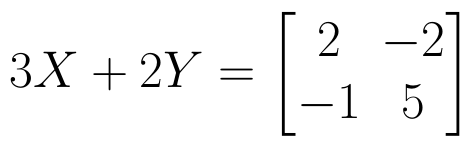(View Answer Video)

Question 5 : Find the value of y,  from the equation: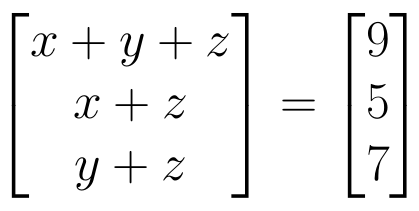. (View Answer Video)

### Application of Integrals

Question 1 : Find the area of smaller region bounded by the ellipse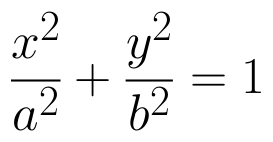and the line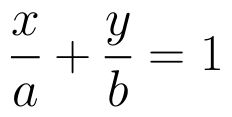. (View Answer Video)

Question 2 : Using integration, find the area bounded by the tangent to the curve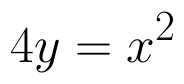at the point (2, 1) and the lines whose equations are x = 2y and x = 3y - 3. (View Answer Video)

Question 3 : Find the area of the smaller part of the circle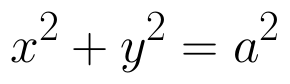cut off by the line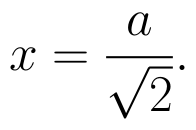(View Answer Video)

Question 4 : Find the area of the smaller region bounded by the ellipse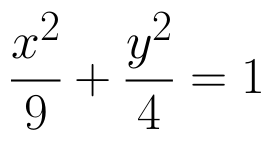and the line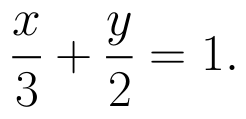(View Answer Video)

Question 5 : Find the area of the region bounded by the curve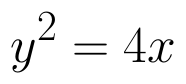and the line x = 3. (View Answer Video)